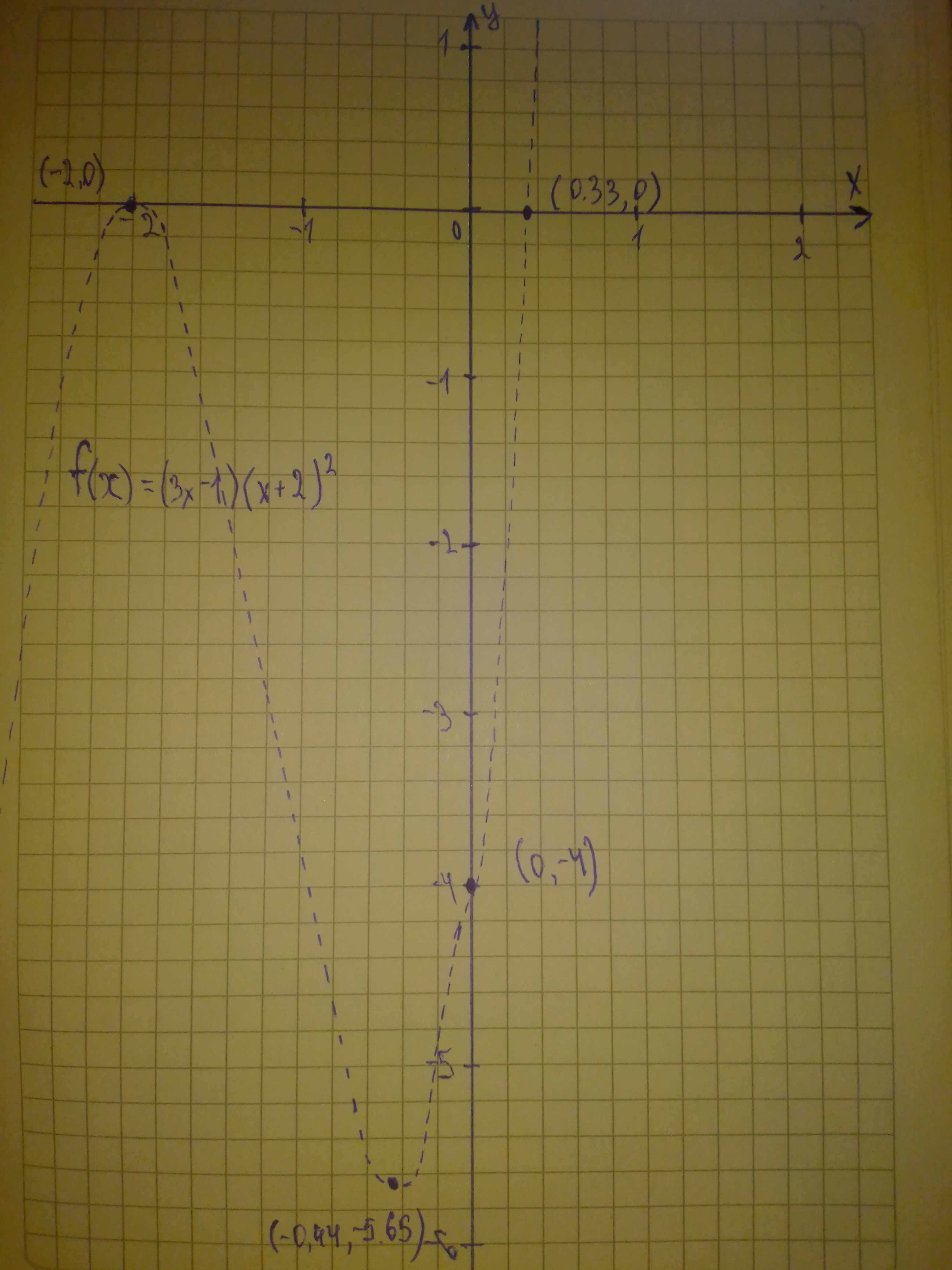Ask question

# Graph each polynomial function. Factor first if the expression is not in factored form. f(x)=(3x-1)(x+2)^{2}# Graph each polynomial function. Factor first if the expression is not in factored form. f(x)=(3x-1)(x+2)^{2}

Question
Polynomial graphsasked 2021-01-13
Graph each polynomial function. Factor first if the expression is not in factored form.
$$\displaystyle{f{{\left({x}\right)}}}={\left({3}{x}-{1}\right)}{\left({x}+{2}\right)}^{{{2}}}$$

## Answers (1)2021-01-14
Step 1
$$\displaystyle{f{{\left({x}\right)}}}={\left({3}{x}-{1}\right)}{\left({x}+{2}\right)}^{{{2}}}$$ The function in factored form
The function has two zeros $$\displaystyle{\frac{{{1}}}{{{3}}}}$$ and -2ZSK
So, the graph of f(x) crosses the x-axis at $$\displaystyle{\left({\frac{{{1}}}{{{3}}}},{0}\right)}{\quad\text{and}\quad}{\left(-{2},{0}\right)}$$
To find the y-intercept, substitute 0 for x in f(x)
$$\displaystyle{f{{\left({x}\right)}}}={\left({3}{x}-{1}\right)}{\left({x}+{2}\right)}^{{{2}}}$$
$$\displaystyle{f{{\left({0}\right)}}}={\left({3}{\left({0}\right)}-{1}\right)}{\left({0}+{2}\right)}^{{{2}}}$$ Substitute 0 for x
$$\displaystyle={\left(-{1}\right)}{\left({2}\right)}^{{{2}}}$$ Simplify
$$\displaystyle=-{4}$$
So, the function f(x) crosses the y-axis at $$\displaystyle{\left({0},-{4}\right)}$$
Step 2
$$\displaystyle{3}{x}\cdot{\left({x}\right)}^{{{2}}}={3}{x}^{{{3}}}$$
The leading coefficient is 3
Since the leading coefficient is positive and the function f(x) of degree 3 (odd degree)
So, the end behavior is
$$\displaystyle{x}\rightarrow\infty,{f{{\left({x}\right)}}}\rightarrow\infty$$
$$\displaystyle{x}\rightarrow-\infty,{f{{\left({x}\right)}}}\rightarrow-\infty$$
See the graph below### Relevant Questionsasked 2021-01-22
Graph each polynomial function. Factor first if the expression is not in factored form.
$$\displaystyle{f{{\left({x}\right)}}}={x}^{{{2}}}{\left({x}+{1}\right)}{\left({x}-{1}\right)}$$asked 2021-02-12
Graph each polynomial function. Factor first if the expression is not in factored form. $$\displaystyle{f{{\left({x}\right)}}}={x}^{{{2}}}{\left({x}-{5}\right)}{\left({x}+{3}\right)}{\left({x}-{1}\right)}$$asked 2021-01-10
Graph each polynomial function. Factor first if the expression is not in factored form. $$\displaystyle{f{{\left({x}\right)}}}={\left({4}{x}+{3}\right)}{\left({x}+{2}\right)}^{{{2}}}$$asked 2020-11-20
Graph each polynomial function. Factor first if the expression is not in factored form.
$$\displaystyle{f{{\left({x}\right)}}}={2}{x}^{{{3}}}{\left({x}^{{{2}}}−{4}\right)}{\left({x}−{1}\right)}$$asked 2020-11-08

Graph each polynomial function. Factor first if the expression is not in factored form. $$f(x)=x^{3}\ +\ 3x^{2}\ -\ 13x\ -\ 15$$asked 2021-05-16
Consider the curves in the first quadrant that have equationsy=Aexp(7x), where A is a positive constant. Different valuesof A give different curves. The curves form a family,F. Let P=(6,6). Let C be the number of the family Fthat goes through P.
A. Let y=f(x) be the equation of C. Find f(x).
B. Find the slope at P of the tangent to C.
C. A curve D is a perpendicular to C at P. What is the slope of thetangent to D at the point P?
D. Give a formula g(y) for the slope at (x,y) of the member of Fthat goes through (x,y). The formula should not involve A orx.
E. A curve which at each of its points is perpendicular to themember of the family F that goes through that point is called anorthogonal trajectory of F. Each orthogonal trajectory to Fsatisfies the differential equation dy/dx = -1/g(y), where g(y) isthe answer to part D.
Find a function of h(y) such that x=h(y) is the equation of theorthogonal trajectory to F that passes through the point P.asked 2021-06-04
Determine whether $$F(x)=5x^{4}-\pi x^{3}+\frac{1}{2}$$ is a polynomial. If it is, state its degree. If not, say why it is not a polynomial. If it is a polynomial, write it in standard form. Identify the leading term and the constant term.asked 2021-05-13
Factor each polynomial. If a polynomial cannot be factored, write prime. Factor out the greatest common factor as necessary:
$$3x^{2}+4x-7$$asked 2021-04-20
Is the algebraic expression a polynomial? If it is, write the polynomial in standard form:
$$\displaystyle{2}{x}+{3}{x}^{{{2}}}-{5}$$asked 2021-06-03
Determine whether the following function is a polynomial function. If the function is a polynomial​ function, state its degree. If it is​ not, tell why not. Write the polynomial in standard form. Then identify the leading term and the constant term.
$$g(x)=3-\frac{x^{2}}{4}$$
...